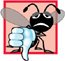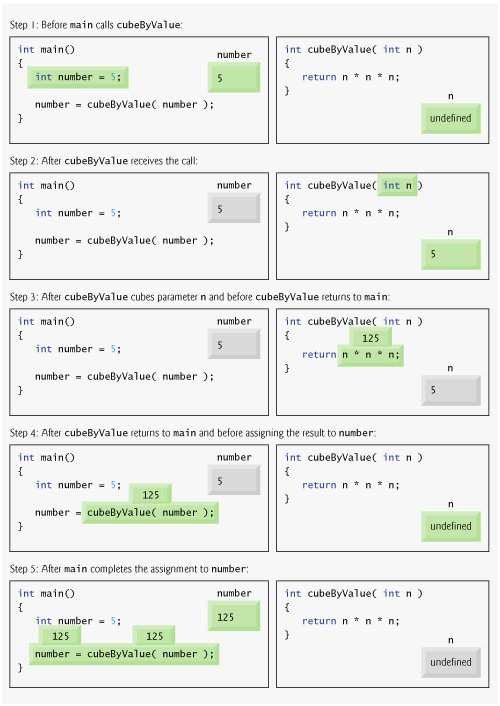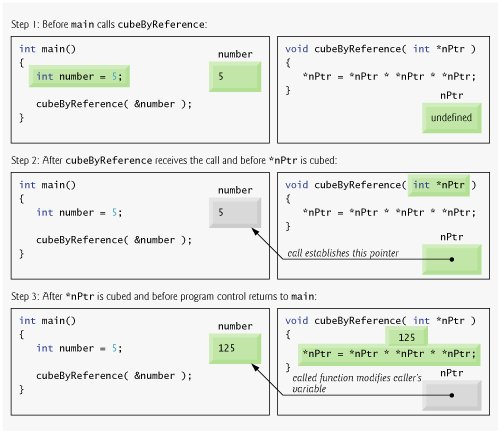# Passing Arguments to Functions by Reference with Pointers

There are three ways in C++ to pass arguments to a functionpass-by-value, pass-by-reference with reference arguments and pass-by-reference with pointer arguments. Chapter 6 compared and contrasted pass-by-value and pass-by-reference with reference arguments. In this section, we explain pass-by-reference with pointer arguments.

As we saw in Chapter 6, return can be used to return one value from a called function to a caller (or to return control from a called function without passing back a value). We also saw that arguments can be passed to a function using reference arguments. Such arguments enable the called function to modify the original values of the arguments in the caller. Reference arguments also enable programs to pass large data objects to a function and avoid the overhead of passing the objects by value (which, of course, requires making a copy of the object). Pointers, like references, also can be used to modify one or more variables in the caller or to pass pointers to large data objects to avoid the overhead of passing the objects by value.

In C++, programmers can use pointers and the indirection operator (*) to accomplish pass-by-reference (exactly as pass-by-reference is done in C programs, because C does not have references). When calling a function with an argument that should be modified, the address of the argument is passed. This is normally accomplished by applying the address operator (&) to the name of the variable whose value will be modified.

As we saw in Chapter 7, arrays are not passed using operator &, because the name of the array is the starting location in memory of the array (i.e., an array name is already a pointer). The name of an array, arrayName, is equivalent to &arrayName[ 0 ]. When the address of a variable is passed to a function, the indirection operator (*) can be used in the function to form a synonym for the name of the variablethis in turn can be used to modify the value of the variable at that location in the caller's memory.

Figure 8.6 and Fig. 8.7 present two versions of a function that cubes an integercubeByValue and cubeByReference. Figure 8.6 passes variable number by value to function cubeByValue (line 15). Function cubeByValue (lines 2124) cubes its argument and passes the new value back to main using a return statement (line 23). The new value is assigned to number (line 15) in main. Note that the calling function has the opportunity to examine the result of the function call before modifying variable number's value. For example, in this program, we could have stored the result of cubeByValue in another variable, examined its value and assigned the result to number only after determining that the returned value was reasonable.

Figure 8.6. Pass-by-value used to cube a variable's value.

``` 1 // Fig. 8.6: fig08_06.cpp
2 // Cube a variable using pass-by-value.
3 #include
4 using std::cout;
5 using std::endl;
6
7 int cubeByValue( int ); // prototype
8
9 int main()
10 {
11 int number = 5;
12
13 cout << "The original value of number is " << number;
14
15 number = cubeByValue( number ); // pass number by value to cubeByValue
16 cout << "
The new value of number is " << number << endl;
17 return 0; // indicates successful termination
18 } // end main
19
20 // calculate and return cube of integer argument
21 int cubeByValue( int n )
22 {
23  return n * n * n; // cube local variable n and return result
24 } // end function cubeByValue
```

 ``` The original value of number is 5 The new value of number is 125 ```

Figure 8.7. Pass-by-reference with a pointer argument used to cube a variable's value.

(This item is displayed on page 409 in the print version)

``` 1 // Fig. 8.7: fig08_07.cpp
2 // Cube a variable using pass-by-reference with a pointer argument.
3 #include
4 using std::cout;
5 using std::endl;
6
7 void cubeByReference( int * ); // prototype
8
9 int main()
10 {
11 int number = 5;
12
13 cout << "The original value of number is " << number;
14
15 cubeByReference( &number ); // pass number address to cubeByReference
16
17 cout << "
The new value of number is " << number << endl;
18 return 0; // indicates successful termination
19 } // end main
20
21 // calculate cube of *nPtr; modifies variable number in main
22 void cubeByReference( int *nPtr )
23 {
24  *nPtr = *nPtr * *nPtr * *nPtr; // cube *nPtr
25 } // end function cubeByReference
```

 ``` The original value of number is 5 The new value of number is 125 ```

Figure 8.7 passes the variable number to function cubeByReference using pass-by-reference with a pointer argument (line 15)the address of number is passed to the function. Function cubeByReference (lines 2225) specifies parameter nPtr (a pointer to int) to receive its argument. The function dereferences the pointer and cubes the value to which nPtr points (line 24). This directly changes the value of number in main.

Common Programming Error 8.5Not dereferencing a pointer when it is necessary to do so to obtain the value to which the pointer points is an error.

A function receiving an address as an argument must define a pointer parameter to receive the address. For example, the header for function cubeByReference (line 22) specifies that cubeByReference receives the address of an int variable (i.e., a pointer to an int) as an argument, stores the address locally in nPtr and does not return a value.

The function prototype for cubeByReference (line 7) contains int * in parentheses. As with other variable types, it is not necessary to include names of pointer parameters in function prototypes. Parameter names included for documentation purposes are ignored by the compiler.

Figures 8.88.9 analyze graphically the execution of the programs in Fig. 8.6 and Fig. 8.7, respectively.

Figure 8.8. Pass-by-value analysis of the program of Fig. 8.6.

(This item is displayed on page 410 in the print version)Figure 8.9. Pass-by-reference analysis (with a pointer argument) of the program of Fig. 8.7.

(This item is displayed on page 411 in the print version)Software Engineering Observation 8.1Use pass-by-value to pass arguments to a function unless the caller explicitly requires that the called function directly modify the value of the argument variable in the caller. This is another example of the principle of least privilege.

In the function header and in the prototype for a function that expects a one-dimensional array as an argument, the pointer notation in the parameter list of cubeByReference may be used. The compiler does not differentiate between a function that receives a pointer and a function that receives a one-dimensional array. This, of course, means that the function must "know" when it is receiving an array or simply a single variable for which it is to perform pass-by-reference. When the compiler encounters a function parameter for a one-dimensional array of the form int b[], the compiler converts the parameter to the pointer notation int *b (pronounced "b is a pointer to an integer"). Both forms of declaring a function parameter as a one-dimensional array are interchangeable.C++ How to Program (5th Edition)
ISBN: 0131857576
EAN: 2147483647
Year: 2004
Pages: 627